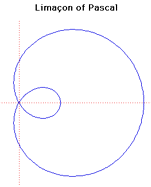A# limaçon of PascalThe limaçon of Pascal is a snail-shaped curve (limaçon is French for "snail"), named by the French mathematician Gilles Roberval after Etienne Pascal, the father of Blaise Pascal. It had been discovered earlier, however; Albrecht Dürer gave a method for drawing it as early as 1525 in his Underweysung der Messung.

The limaçon of Pascal is a special case of an epitrochoid in which the rolling circle and the rolled circle have the same radius, and is also the catacaustic (see caustic curve) and the pedal curve of the circle. It has the quartic Cartesian equation

(x 2 + y 2 – 2rx)2 = k 2(x 2 + y 2)

where r is the radius of the rolling circle or the rolled circle and k is a constant.

Sometimes the term ordinary limaçon is used to describe the curve when the value of k is greater than 0 and less than 1. When k = 0 the curve is a circle and when k = 1 the curve is a cardioid, so the ordinary limaçon is a transitional form between these two. The ordinary limaçon is also the inverse of the ellipse. For values of k > 1, a loop or noose appears in the curve. The inverse of a limaçon with a noose is a hyperbola. In fact, the constant k is the same as the eccentricity for a conic section. When k = 2, the limaçon is also called a trisectrix.